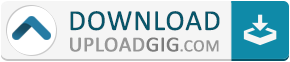#Do not remember me!

### Categories» » » Python Numpy 101 Exercises: Skyrocket your Python skill

## Python Numpy 101 Exercises: Skyrocket your Python skillEnglish | 2020 | ASIN : B08KGP5MKL | 162 Pages | PDF, EPUB, AZW3 | 72.36 MB

Thank you for picking up this book. This book is a practical introduction to "Numpy" for first-time Python users.
You will learn how to write a real program in Python through 101 problems.
The goal is to help students learn to write code that takes full advantage of Numpy's capabilities.

1) People who have learned the basic Python syntax and want to take the next step.

2) If you want to write fast-running, concise Python programs.

3) If you're also a little curious about the mechanics behind deep learning and machine learning.

4) Those who get defensive when they hear the words vector and matrix.

5) Those who want to handle large scale data.

6) Those who want to study a little bit every day

7) If you have started to solve the numpy 100 exercises, but are frustrated

This book starts with "import numpy as np" and lays the foundation for doing things like linear algebra and basic statistics in machine learning.
Programming is often said to be "better to get used to it than to learn it," but if you don't take the time to build an environment to get used to it and get to the point where you can't get to the point
It's not even original. This book includes a link to the executable Google Colaboratory source code so that you can use
You can actually run the code and modify it to solve the problems without the hassle of building an environment.

Although 101 problems and solutions are listed, explanations are omitted for problems that may be unnecessary if you read the source code.
However, explanations are omitted for problems that do not require explanation if you read the source material. If you have any difficulties in understanding the source, please send us an email using Feedback.
I also tweet about supplements and corrections to the book on Twitter (@chqBYIOmA0SYl1F).

01: Import Numpy as np.
02: Check the version of Numpy.
03: Check the type of BLAS used by Numpy.
04: Generate a one-dimensional vector whose size is 10 and whose all elements are 0.
05: Generate a one-dimensional list of all elements of size 10 that are 0.
06: Assign the list of 04 and 05 to be indexed as values, and print the address of each element by id().
07: Get Numpy's np.dot document from the command line.
08: After defining a vector of all elements 0 of size 10, set the fifth element to 1.
09: Measure the execution speed of Numpy's ndarray and Python list to generate a vector with each element increasing by 1 from 100 to 999999.
11: Generate a 3 x 3 unit matrix.
12: Define a random number array A with 512 x 768 shapes, 12 random number arrays B with 768 x 768 shapes (B1 - B12), and a random number array C with 768 x 2, and output the results of dot product operations on all of them in sequence.
13: Create a matrix of 10 x 10 SHAPE random numbers and find the maximum and minimum values.
14: Generate an array of random numbers of size 30 and calculate the average value.
15: Generate a two-dimensional array that is surrounded by 1s on all sides and 0s in the middle.
16: Represent the initial state of Othello in Numpy.
17: Surround a 5 x 5 matrix whose elements are all 0's with 1's around it.
18: Surround a 7 x 7 matrix with all 0 elements by 1. However, write it in a different way than in No. 17.
19: Create a 5 x 5 0 matrix and put 1,2,3,4,5 on the diagonal.
20: Create an 8 x 8 matrix and represent the checkerboard pattern by 01.
21: Given an array of the form (6,6,6), find the index (x,y,z) of the 100th element.
...
101
???Name:* E-Mail: Security Code: *